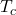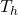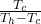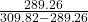Question

A Carnot air conditioner takes energy from the thermal energy of a room at 61°F and transfers it as heat to the outdoors, which is at 98°F. For each joule of electric energy required to operate the air conditioner, how many joules are removed from the room?

1.14.07 units of joules is removed for each joules of electricity used

Explanation:

The heated room temperature= 61 °F

The outside temperature= 98 °F

For conversion from fahrenheit to kelvin we use the equation

(32°F − 32) × 5/9 + 273.15= 289.26= 309.82

For air conditioning,

COP =COP == 14.07

This means that 14.07 units of joules is removed for each joules of electricity used